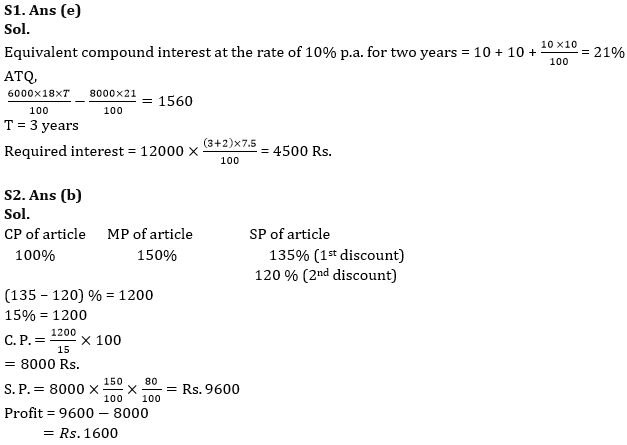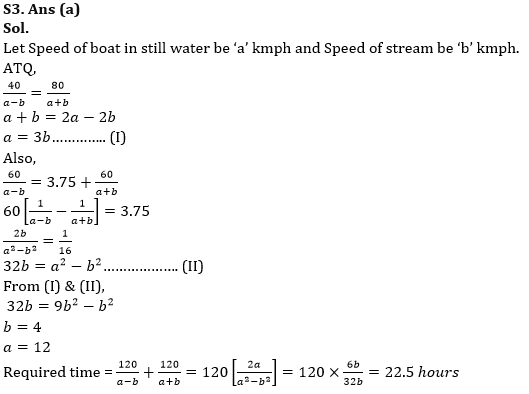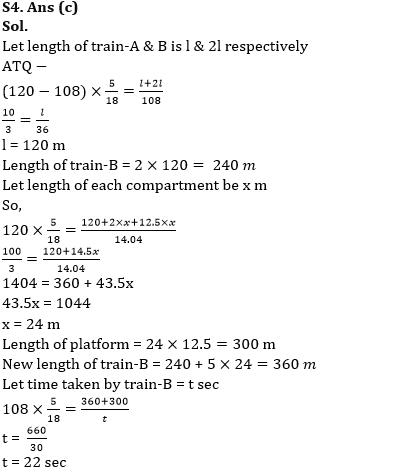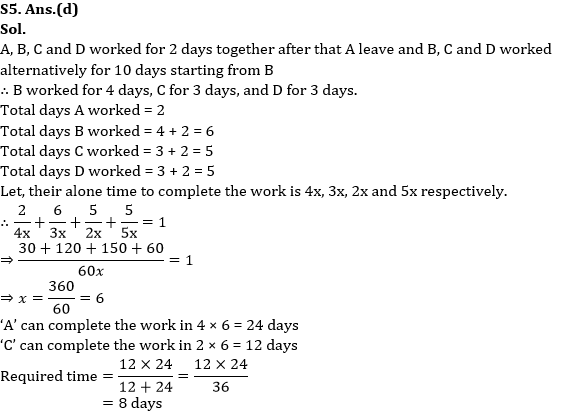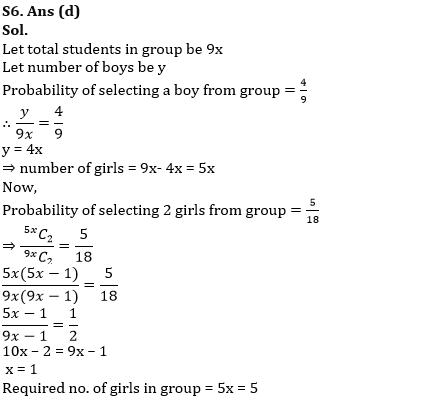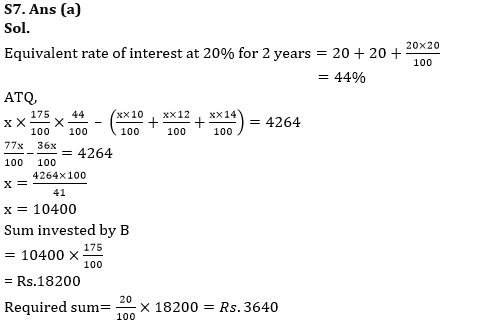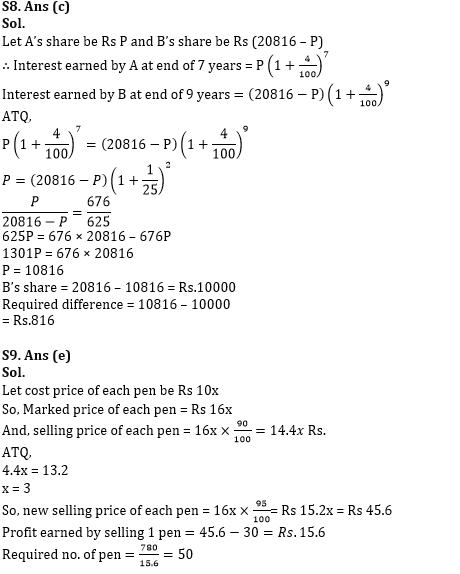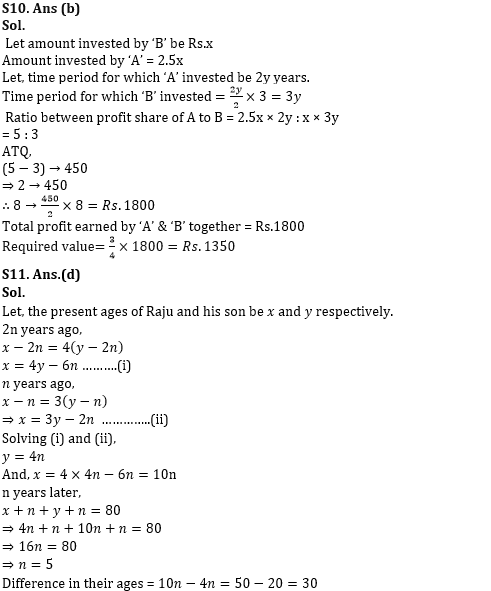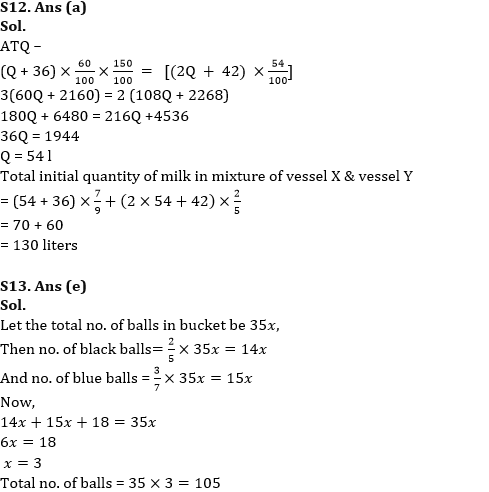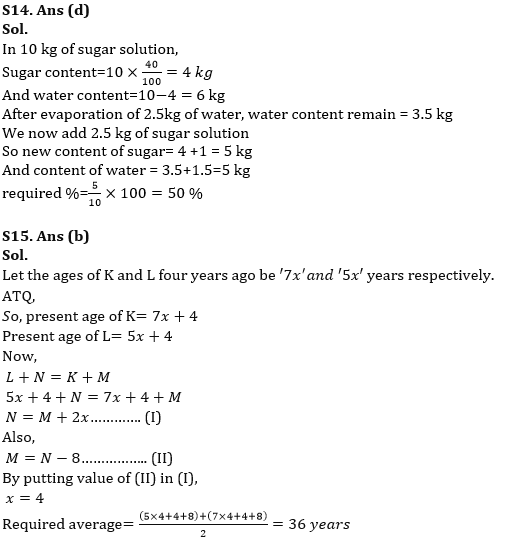Latest Banking jobs   »

# Quantitative Aptitude Quiz For RBI Grade B/ ECGC PO/ SIDBI Grade A Prelims 2022- 19th April

Q1. Rohan invested Rs.6000 at 18% p.a. on simple interest for T years, while Shyam invested Rs.8000 at 10% p.a. compounding annually for two years. Rohan received Rs.1560 more as interest than Shyam. If Shyam invested Rs.12000 at 7.5% p.a. on simple interest for (T+2) years, then he gets total interest of Rs. ______.
(a)6000
(b)4000
(c)5500
(d)5000
(e)4500

Q2. A shopkeeper marked up the price of an article by 50%. If he increases the discount from 10% to 20%, the profit would decrease by Rs.1200, then find how much profit shopkeeper would earn if he gives a discount of 20% on the marked price?(in Rs.)
(a)2000
(b)1600
(c)2400
(d)1800
(e)4200

Q3. A boat can cover 40 km upstream in same time in which it can cover 80 km downstream and time taken by boat to cover 60 km upstream is 3.75 hours more than time taken by it to cover same distance in downstream. Find in how much time boat can cover a distance of 120 km upstream and downstream both? (in hours)
(a)22.5
(b)15.5
(c)27.5
(d)12.5
(e)None of these

Q4. Ratio between length of train-A & train B is 1: 2 and speed of train-A & train B is 120 km/hr & 108 km/hr respectively. Both trains running in same direction and cross each other in 108 sec. If two compartments were added in train-A then it can cross a platform of length 12.5 times of length of one compartment in 14.04 sec, then find the time taken by train-B to cross that same platform, if five new compartments were added in that same train only?
(a)36 sec
(b)24 sec
(c)22 sec
(d)28 sec
(e)45 sec

Q5. Four persons started to do a work together. ‘A’ works only in starting two days after that B, C and D works alternately starting from B. Ratio of time taken by A, B, C and D, if they work alone is 4 : 3 : 2 : 5. If the work is completed in 12 days then in how many days A and C can complete the work if they work together ?
(a) 6 days
(b) 12 days
(c) 10 days
(d) 8 days
(e) None of these

Q6. The probability of selecting 2 girls from a group of students is 5/18 and probability of selecting a boy from same group is 4/9. Find total number of girls in the group?
(a)2
(b)3
(c)4
(d)5
(e)1

Q7. A invested a sum in scheme ‘P’ on SI per annum at the ratio of 10% for first year, 12% for second year and 14% for third year and B invested 75% more sum than A in scheme ‘Q’ on C.I. per annum at the rate of 20% per annum for two years. If B got Rs.4264 interest more than interest earned by A, then find 20% of sum invested by B? (in Rs.)
(a)3640
(b)4210
(c)3220
(d)4440
(e)None of these

Q8. A sum of Rs.20816 was divided between A and B in such a way that interest earned by A at the end of 7 years was equal to interest earned by B at the end of 9 years when both invested there share at CI at 4% p.a. Find A’s share is how much more than the B’s share?
(a)1184
(b)786
(c)816
(d)932
(e)1024

Q9. A seller markup price of each pen by 60% above its cost price and allows a discount of 10% thereby making a profit of Rs.13.2 on each pen. When he sells each pen at only 5% discount, then ________pens he needs to sell in order to earn overall profit of Rs.780.
(a)125
(b)25
(c)75
(d)100
(e)50

Q10. ‘A’ and ‘B’ started a business together. Amount invested by ‘A’ is 250% of amount invested by ‘B’. Ratio between time period of investment of ‘A’ to ‘B’ is 2: 3. Find 3/4th of
(a)1800
(b)1350
(c)2250
(d)2850
(e)3200

Q11. 2n years ago, the age of Raju was four times that of his son and n years ago, the age of Raju was thrice that of his son. If n years later, the sum of the ages of Raju and his son will be 80 years, then the difference in the ages of Raju and his son is
(a) 20 years
(b) 40 years
(c) 24 years
(d) 30 years
(e) 35 years

Q12. Vessel X contains (Q + 36) liter mixture of milk & water in the ratio of 7: 2, while vessel Y contains (2Q + 42) liter mixture of milk & water in the ratio of 2 : 3. If 40% & 46% liter mixture from vessel X and Y taken out respectively, then mixture remaining in vessel Y is 150% of mixture remaining in vessel X. Find the total initial quantity of milk in mixture of vessel X & vessel Y together? (in liters)
(a)130
(b)180
(c)90
(d)150
(e)210

Q13. In a bucket there are different colored balls of black, blue and white colors. The probability of selecting one black ball out of the total ball is 2/5 and the probability of selecting one blue ball out of the total ball is 3/7.The number of white balls in the bag is 18. Find total number of balls in the bucket?
(a)135
(b)80
(c)120
(d)90
(e)105

Q14. A sugar solution has 60% water. If 2.5 kg of water evaporates from 10 kg of the solution and 2.5 kg of initial sugar solution is added again to remaining 7.5 kg of the solution, then find the percentage of sugar in this new solution?
(a)100%
(b)125%
(c)75%
(d)50%
(e)25%

Q15. Four year ago, ratio of age of K and L is 7: 5 and sum of present age of L and N is equal to the sum of present age of K and M. If M is eight years younger than N, then find the average of present age of L and K 8 years hence? (in years)
(a)35
(b)36
(c)20
(d)15
(e)10

Solutions